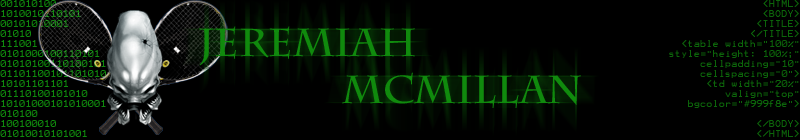# Methods

## What is a method?

• A method is a piece of Java code packaged up so you can reuse it.
• Usually, a method will take some input and give some output
• System.out.println() is an example of a method
• Using a method (calling a method) always requires parentheses

## Idea of a method

• Methods allow you to package up some code to run over and over
• Methods usually take some input (like numbers or Strings) so that they can be customized
• Methods often give back an answer (like the square root of a number)

## Method Stucture

accessModifier returnType methodName(parameters)

### Access Modifier

Access modifiers are either public, private, protected, or default

• public - Any program can use the method if it's public
• private - Only the class containing the method can use the method
• protected - Only classes in the same package can access the method. If the class is sub classed, then the method can be access regardless of the package.
• default - Default access occurs when you leave the access modifer blank.

### Return Type

Methods can return primitives (int, double, boolean, char) ,objects, or void

• void - void method do not return a value. Void methods only do something. If you try to save the value they return, there will be a compiler error
• protected - Only classes in the same package can access the method. If the class is sub classed, then the method can be access regardless of the package.
• default - Default access occurs when you leave the access modifer blank.

## Simple method example

Given two integers, find the bigger:

public int max(int a, int b)
{

if( a > b )
return a;
else
return b;

}

### Why Object Reference Methods

• object methods are methods connected to a particular object
• They perform a simple or specific task

Proper syntax for calling a static method gives first the reference variable (of the instance of class) , a dot, the name of the method, then the arguments

ObjectReferenceVariable.nameMethod(arg1, arg2, arg3);

### Examples

String s1 = new String("Hello");
System.out.println("The lenght is " + s1.length());

output
The length is 5

Random gen = new Randon();
int num = gen.nextInt(10); //num contains 9

## Simple static method example

Given two integers, find the smaller:

public static int min(int a, int b)
{

if( a < b )
return a;
else
return b;

}

### Why Static

• static methods are methods not connected to a particular object
• They perform a simple or specific task

Proper syntax for calling a static method gives first the name of the class the method is in, a dot, the name of the method, then the arguments

ClassName.MethodName(arg1, arg2, arg3);

### Examples

int num = Math.abs(-3); // num is equal to 3
int high = Math.max(4,3); // high = 4

## Scanner ClassCredit: A Slides are modified with permission from Barry Wittman at Elizabethtown College